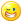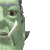## Welcome to the Second Life Forums Archive

These forums are CLOSED. Please visit the new forums HERE

# Bitwise rotation

Eric Quine
Registered User
Join date: 5 Jun 2006
Posts: 3
07-04-2007 10:48
CODE
`string RotateBits(string bits, integer amount, string direction){    integer x;    integer size = llStringLength(bits) - 1;    string value;    if(direction == "RIGHT")    {        for (x = 0; x < amount; x++)        {            string val = llGetSubString(bits, size, size) +            llDeleteSubString(bits, size, size);            value = val;            bits = value;}        }    if(direction == "LEFT")    {        for (x = 0; x < amount; x++)        {            string a = llGetSubString(bits, 0, 0);            string b = llDeleteSubString(bits, 0, 0);            value = b+a;            bits = value;}        }    return value;}string RotateMultipleBits(string bits, integer stride, integer amount, string direction){    integer length = llStringLength(bits);    integer x;    string value;        for ( x = 0; x < length; x+=stride )    {        string b = llGetSubString(bits, x, x+stride-1);        string v = RotateBits(b, amount, direction);        value += v;    }    return value;}`

was playing about and figured this could be a little useful for someone trying to create an encryption, rotation is rather simple and you can easily recover the original bit of info by rotating it back as many times as you rotated it forward, these functions are for rotating binary but any string you put in will be rotated since its not much of a mathematical function as it is just re formating strings, they work like so:

__________________________________________________

RotateBits("0001", 1 , "RIGHT";

that is saying you want to rotate "0001" one time to the right,

any amount of bits put into this function will work.

it will rotate a nybble, or a byte, and so on so fourth.

__________________________________________________

RotateMultipleBits("1101111010101101", 4, 1, "RIGHT";

that is saying you want to rotate every four bits in the binary 1 time to the right,
more easily looked at like this:

1101 1110 1010 1101 each section one time to the right equals this 1110 0111 0101 1110.

(do not put spaces in the bits you want to rotate, that was just easier to look at.)

__________________________________________________

this is the format for each

RotateBits(string bits, integer amount, string direction)

bits is what you are rotating, amount is by how much, and direction is which way "LEFT or RIGHT"

RotateMultipleBits(string bits, integer stride, integer amount, string direction)

its almost the same as the last, except for stride, which is how many bits at a time to rotate.

__________________________________________________

these both return strings.

you need to have both for the RotateMultipleBits function to work since they are dependent of one another.

enjoy :3
Nada Epoch
The LibrarianJoin date: 4 Nov 2002
Posts: 1,423
Discussion Thread
07-12-2007 22:23
/54/51/197200/1.html
_____________________
i've got nothing.